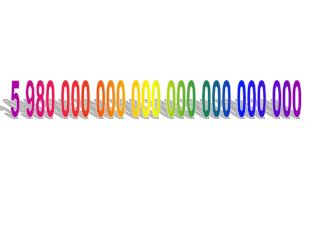DownloadDownload Presentation5 980 000 000 000 000 000 000 000

5 980 000 000 000 000 000 000 000

Download Presentation5 980 000 000 000 000 000 000 000

- - - - - - - - - - - - - - - - - - - - - - - - - - - E N D - - - - - - - - - - - - - - - - - - - - - - - - - - -
Presentation Transcript

1. 5 980 000 000 000 000 000 000 000

2. Scientific Notation • Scientific Notation is a short-hand way of writing down really big number, or really small numbers. • The mass of the earth is: 5 980 000 000 000 000 000 000 000 • Saying this number is not an easy task. • Writing these zero’s is not very convenient (or fun) • To help us we use Scientific Notation

3. Scientific Notation Numbers expressed in scientific MUST be expressed as a product of: • a number greater or equal to one, but less than ten And • A power of ten

4. Are these Numbers in Scientific Notation? • 33.1 x 104 • 11 x 102 • 5.3 x 108 • 9.9 x 1011 • 0.3 x 102 • 5 x 107 • 2.3108 x 105

5. What is 561 000 000 000 in Scientific Notation? • 1. Place a decimal point after the first non-zero digit(giving you a number greater or equal to 1 but less than 10) • 5.61 000 000 000 • Drop the trailing zeros • 5.61 • Count the number of places(boinks) the decimal was moved. • 561 000 000 000 • Express this number as an exponent of 10 • 5.61 x 1011

6. What is 420 000 000 in Scientific Notation? • 1. Place a decimal point after the first non-zero digit(giving you a number greater or equal to 1 but less than 10) • 4.20 000 000 • Drop the trailing zeros • 4.2 • Count the number of places (boinks) the decimal was moved. • 420 000 000 • Express this number as an exponent of 10 • 4.2 x 108

7. What is 580 000 in Scientific Notation? • 1. Place a decimal point after the first non-zero digit(giving you a number greater or equal to 1 but less than 10) • 5.80 000 • Drop the trailing zeros • 5.8 • Count the number of places (boinks) the decimal was moved. • 580 000 • Express this number as an exponent of 10 • 5.8 x 105

8. What is the Value of Each Exponent? • 310 000 = 3.1 x 10? • 81 000 000 = 8.1 x 10? • 5.3 x 108 = ? • 9.9 x 1011 = ? • 5.3 x 102 = ? • 9 x 107 = ? • 2.3108 x 105 = ?